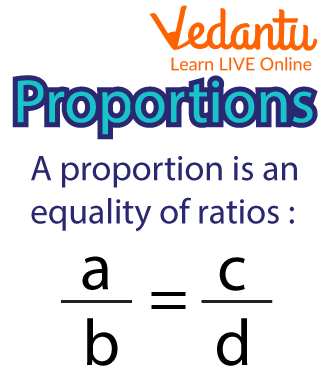Courses
Courses for Kids
Free study material
Free LIVE classes
More

# What is Proportion?LIVE
Join Vedantu’s FREE Mastercalss

## An Overview of Proportion

An equation known as proportion shows that the two ratios given are equal to one another. In other words, the proportion declares that the two ratios or fractions are equal. In terms of proportion, two sets of given numbers are said to be directly proportional if they increase or decrease in the same ratio.

Symbol of Proportion: The proportion is denoted by the symbol ‘=’ or ‘::’.

Example: When two ratios are equal, they are said to be in proportion. For instance, the time it takes a train to travel 60 kilometres per hour is equal to the time it needs to travel 300 kilometres in 5 hours. For example, 300 km/5 hours at 50 km/h. There are two types of proportion:

• Direct Proportion

• Indirect Proportion

## Basics of Proportion

Let us first consider a,b,c and d as four quantities of proportion. So, we may write the proportion as a:b::c:d or a:b=c:d. Let us start explaining the proportions from these representations. Here:

• a and d are the first and fourth term, respectively, known as extremes terms.

• b and c are the second and third term, respectively, known as the mean terms.

• Product of extremes = Product of mean.Proportion

### Fourth Proportion

In the representation a:b=c:d, the letter d is known as the fourth proportion to a,b and c. For example, if 6,7 and 8,9 are in proportion, then 9 will be the fourth proportion to 6, 7 and 8.

### Fourth Proportional Formula

If we consider a, b, c and d are in proportion, then a:b::c:d. To calculate the fourth term that is d,

$\dfrac{a}{b} = \dfrac{c}{d}$

Therefore,

$d = \dfrac{{b \times c}}{a}$

### Mean Proportion

Here in the proportion a:b::c:d, b and c are known as the mean terms. Thus, the square root of the product of these two is the mean proportional between the two terms of a ratio in a proportional.

### Third Proportion

The second term of the mean terms serves as the third proportional of a proportion. If a:b = c:d, for instance, the term "c" is the third proportional to "a" and "b."

### How to Find Fourth Proportional

In the above example, we have seen the explanation and formula for the calculation of the fourth proportion. Here let us understand it with the help of examples:

1. Find the fourth Proportion to 10, 20, 30.

Explanation: Let the fourth proportion to 10, 20 and 30 be x.

Then, 10:20:: 30: x

$\Rightarrow 10 \times x = 20 \times 30$(Product of extreme= product of mean)

$\Rightarrow x = \dfrac{{20 \times 30}}{{10}}$

$\Rightarrow x = 60$

Hence, the fourth proportion to 10, 20, 30 is 60.

### Summary

The equality of two ratios is referred to as proportion. The ratio symbol (::) is used to denote proportions, which aid in solving problems involving ambiguous quantities. To put it another way, proportion is an expression or equation that shows that two ratios or fractions are equal.

### Solved Questions

1.What will be the fourth term if the first three terms of the proportion are 6, 12, and 24.

Ans: Let the fourth term be x.

Then, 6:12 :: 24:x

$\Rightarrow x = \dfrac{{12 \times 24}}{6}$

$\Rightarrow x = 48$

Thus, the fourth term is 48.

2.Find out if the ratios 4:8 and 12:24 are in proportion.

Ans: For the given ratio :

$\Rightarrow \dfrac{4}{8} = \dfrac{1}{2} = 0.5$ & $\dfrac{{12}}{{24}} = \dfrac{1}{2} = 0.5$

Hence, both the ratios are in proportion.

3.Find the value of x so that the given four numbers are in proportion:

4, 10, 24, and x

Ans: So here, the product of mean = product of extreme

$\Rightarrow x = \dfrac{{10 \times 24}}{4} = 60$

Hence, the value of x is 60.

### Learning by Doing

1. Find out if the ratios 9:36 and 4:24 are in proportion.

2. What is the proportion?

Last updated date: 26th Sep 2023
Total views: 90.9k
Views today: 1.90k

## FAQs on What is Proportion?

1. A sweet dish required 3 kgs of sugar for every 6 kgs of flour. If 60kgs of this sweet has to be prepared, how much sugar is required?

Let the quantity of sugar required be x kgs.

3 kgs of sugar added to 6 kgs of flour constitutes.

The ratio of sugar and flour will be constant

Hence, The ratio of sugar and flour in the recipe $= \frac{3}{6} = \frac{1}{2} = 1:2$

To prepare 60 kg of sweet, the required sugar will be :

$\Rightarrow \dfrac{2}{4} = \dfrac{x}{{40}}$$x = 20$. Therefore, 20 kgs of sugar is required for 60 kgs of sweet.

2. Find the fourth proportional to 8, 24 and 40?

Let the fourth proportion be x.

Then, 8:24::40:x

$\Rightarrow x = \dfrac{{24 \times 40}}{8} = 120$

Hence, the value of the fourth proportion is 120.

3. If the mean proportion is 40 and one extreme is 10. How will you find the other extreme?

Let the other extreme be x.

Then, product of mean = product of extreme

X = product of mean /one extreme

Then, $\dfrac{{40}}{{10}} = 4$

The other extreme is  4.